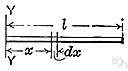# moment of inertia

(redirected from Principal moments of inertia)
Also found in: Thesaurus, Encyclopedia.
Related to Principal moments of inertia: Mass moment of inertia

## moment of inertia

n. pl. moments of inertia
A measure of a body's resistance to angular acceleration, equal to:
a. The product of the mass of a particle and the square of its distance from an axis of rotation.
b. The sum of the products of each mass element of a body multiplied by the square of its distance from an axis.
c. The sum of the products of each element of an area multiplied by the square of its distance from a coplanar axis.

## moment of inertia

n
(General Physics) the tendency of a body to resist angular acceleration, expressed as the sum of the products of the mass of each particle in the body and the square of its perpendicular distance from the axis of rotation. Symbol: I

## mo′ment of iner′tia

n.
the sum of the products of the mass and the square of the perpendicular distance to the axis of rotation of each particle in a body rotating about an axis.
[1820–30]
ThesaurusAntonymsRelated WordsSynonymsLegend:
 Noun 1moment of inertia - the tendency of a body to resist angular accelerationinertia - (physics) the tendency of a body to maintain its state of rest or uniform motion unless acted upon by an external forcemoment - a turning force produced by an object acting at a distance (or a measure of that force)
Site: Follow: Share:
Open / Close